﻿ Design and Development of an Amplitude Modulator Using Square Law Approach

### Design and Development of an Amplitude Modulator Using Square Law Approach

Folorunso C.O., Akinyemi L.A., Adedoyin M.A.OPEN ACCESSPEER-REVIEWED

## Design and Development of an Amplitude Modulator Using Square Law Approach

Folorunso C.O.1,, Akinyemi L.A.1, Adedoyin M.A.1

1Department of Electronic and Computer Engineering, Lagos State University, Lagos Nigeria

### Abstract

This paper discusses a simple, reliable and cheap way of achieving Amplitude Modulation (AM) using a square law method. Amplitude modulation is a technique used in electronic communication most commonly for transmitting information via a . This is achieved by varying the strength of the carrier signal in relation to the information being sent. Hence, the changes in the maximum and minimum values of carrier signal result to the varying modulating index at 0.5 ms/Div sweep on the Oscilloscope screen in which the respective waveforms were displayed ranging from 47.4% to 100% at 1 KHz and 200 KHz modulating and Carrier frequencies respectively.

### At a glance: Figures

123
Prev Next

• C.O., Folorunso, Akinyemi L.A., and Adedoyin M.A.. "Design and Development of an Amplitude Modulator Using Square Law Approach." International Transaction of Electrical and Computer Engineers System 2.1 (2014): 39-43.
• C.O., F. , L.A., A. , & M.A., A. (2014). Design and Development of an Amplitude Modulator Using Square Law Approach. International Transaction of Electrical and Computer Engineers System, 2(1), 39-43.
• C.O., Folorunso, Akinyemi L.A., and Adedoyin M.A.. "Design and Development of an Amplitude Modulator Using Square Law Approach." International Transaction of Electrical and Computer Engineers System 2, no. 1 (2014): 39-43.

 Import into BibTeX Import into EndNote Import into RefMan Import into RefWorks

### 1. Introduction

A radio signal can be generated by causing an electromagnetic disturbance and making suitable arrangements for this disturbance to be propagated in free space. The equipment normally used for creating the disturbance is the transmitter, and the transmitter antenna ensures the efficient propagation of the disturbance in free space. To detect the disturbance, one needs to capture some finite portion of the electromagnetic energy and convert it into a form which is meaningful to one of the human senses. The equipment used for this purpose is, of course, a receiver. The energy of the disturbance is captured using an antenna and an electrical circuit then converts the disturbance into an audible signal.

Assuming for a moment that the transmitter propagated a completely arbitrary signal (that is, the signal contained all frequencies and all amplitudes). Then no other transmitter can operate in free space without severe interference because free space is a common medium for the propagation of all electromagnetic waves. However, if there is a restriction to each transmitter to one specific frequency (that is, continuous sinusoidal waveforms) then interference can be avoided by incorporating a narrow-band filter at the receiver to eliminate all other frequencies except the desired one. Such a communication channel would work quite well except that its signal cannot convey information since a sinusoid is completely predictable and information, by definition, must be unpredictable.

Human beings communicate primarily through speech and hearing. Normal speech contains frequencies from approximately 100 Hz to approximately 5 kHz and a range of amplitudes starting from a whisper to very loud shouting. An attempt to propagate speech in free space comes up against two very severe obstacles. The first is similar to that of the transmitters discussed earlier, in which they interfere with each other because they share the same medium of propagation. The second obstacle is due to the fact that low frequencies, such as speech, cannot be propagated efficiently in free space whereas high frequencies can. Unfortunately, human beings cannot hear frequencies above 20 kHz which is, in fact, not high enough for free space transmission. However, if there is a way one can arrange to change some property of a continuous sinusoidal high-frequency source in accordance with speech, then the prospects for effective communication through free space become a distinct possibility. Changing some property of a (high-frequency) sinusoid in accordance with another signal, for example speech is called modulation. It is possible to change the amplitude of the high-frequency signal, called the carrier, in accordance with speech and/or music. The modulation is then called amplitude modulation or AM for short. It is also possible to change the phase angle of the carrier, in this case phase modulation (PM), or frequency modulation (FM) results.

### 2. Related Works

In the past, efforts had been made in different modulation schemes to carry out research on various schemes. Notably among the researchers and their corresponding work are numerated below:

According to Alexander S. et al (2009) who worked on amplitude modulation and demodulation of chaotic signals, it was shown that the use of phase – chaotic signal and coherent receiver gives better results for the demodulated signal with a value signal to disturbance ratio (S/D) was approximately 77 dB .

More so, Hui I. Hsieh and Kourosh Saberi (2010) also researched on the detection of sinusoidal amplitude modulation in logarithmic frequency sweeps across wide regions of the spectrum. The AM-detection thresholds were measured as a function of signal modulation rate and Carriers sweep frequency region. Therefore, striking results were obtained in terms thresholds ranging from – 8 dB for an AM rate at 8 Hz to -30 dB at 128 Hz .

Additionally, Dick Bowdler (2010) carried out research on amplitude modulation of wind turbine noise. It was shown by the analysis that modulation is due to a combination of tower shadows effects as the blades pass the tower plus the predominate radiation of noise into some directions in the right order. Two distinct mechanisms were adapted and fantastic results were equally obtained .

All the methods employed above were expensive and difficult to implement, hence the need for the square-law Amplitude Modulation. This is a novel way of achieving Amplitude Modulation scheme using auto transformer coupling circuit. This approach demystifies the Amplitude Modulation scheme using simple, affordable and available component for its implementation and performs better than some of the already available methods.

### 3. Methodology

3.1. Overall System Design

The design was based on the square law approach which makes use of a single diode, an inductor, a capacitor and two auto transformers to couple the modulating and carrier signals to the circuit, as depicted in the circuit diagram in Figure 1 below.

When a small alternating voltage vd is applied across a forward – biased diode, the ac diode current id will be a function of this voltage . Expanding this function by the stirling’s or macLaurin’s theorem and retaining up to the second order terms in the series expansion, we have: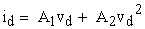(1)

where

A1 and A2 are the series expansion coefficients and id and vd are the instantaneous values of the diode current and the voltage across it.

Let the carrier voltage be given by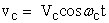(2)

and the modulating voltage by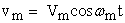(3)

where

vc and vm correspond to the instantaneous values

Vc and Vm are the corresponding peak values, and

ωc and ωm are the respective angular frequencies.

The vd will be given by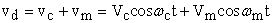(4)

since the two voltage sources are connected in series. Substituting equation (4) in (1) gives: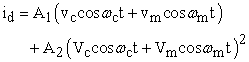or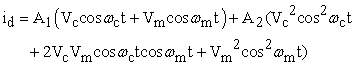(5)

but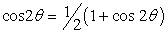(6)

therefore substituting equation (6) in (5) gives: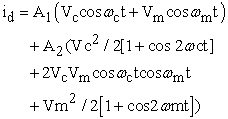(7)

The various frequency components in this equation are:

a.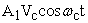= carrier frequency component of the carrier wave

b.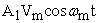= modulating frequency component of the modulating wave

c.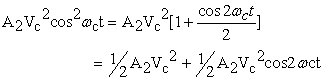There is a direct current (dc) component and a carrier frequency second harmonic component.

d.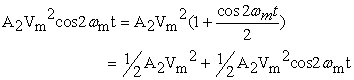There is a dc component and a modulating frequency second – harmonic.

e.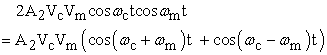The first part is the upper sideband term of frequency given by (ωc + ωm) and the second is the lower sideband term of frequency given by (ωc – ωm).

Thus equation (5) gives rise to six terms of different frequencies in addition to the dc component. The load in this case is a tuned circuit which is adjusted to the carrier frequency ωc. Hence it will respond to a narrow frequency band centered about ωc. If ωm<< ωc, the tuned circuit load will mainly respond to the frequency components (ωc – ωm), ωc and (ωc + ωm). The remaining terms in the circuit expression will not produce enough output voltage across the load.

Hence the desired diode current (mainly effective to produce the output voltage across the load) is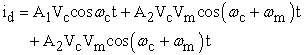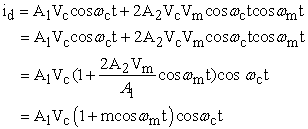(8)

Where the modulation index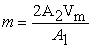.

The modulated output voltage is then given by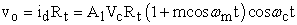(9)

where Rt is the impedance of the tuned circuit at resonance.

The modulation index of the signal can be calculated using equation (10) below: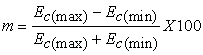(10)
3.2. Circuit Design Parameters
3.2.1. Calculating the Capacitance and Inductance

To calculate the values of the component used, a carrier frequency of 200 KHz and modulating frequency of 1KHz were considered such that the tuned circuit load will mainly respond to the frequency componentConsidering the lower sideband, the resonant frequency of 199 KHz was considered.

Assuming L = 10 mH, C can be calculated thus

Using ω2 = 1 / LC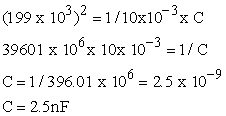Figure 1. Circuit diagram of a Square – Law modulator using audio-transformer coupling

3.2.2. Signal Diode

An IN4148 signal diode was used. Signal diodes are so called because they are commonly found in circuits like those in radios or televisions, to pass very low-power but high-frequency currents. They pass current up to 100 milliamps, and are often used to process information found in electrical signals. By allowing the current to flow only in one direction, they block half of the oscillations of the radio wave, converting the alternating-current wave to direct-current and allowing its varying strength to be read as an audio signal and converted to sound. This diode is a silicon signal type which has a lower resistance and vulnerability to heat.

3.2.3. Audio Transformer

The audio transformers used are those specifically designed for use in audio circuits. They can be used to block radio frequency interference or the DC component of an audio signal, to split or combine audio signals, or to provide impedance matching between high and low impedance circuits, such as between a high impedance tube (valve) amplifier output and a low impedance loudspeaker, or between a high impedance instrument output and the low impedance input of a mixing console.

Such transformers were originally designed to connect different telephone systems to one another while keeping their respective power supplies isolated, and are still commonly used to interconnect professional audio systems or system components.

Being magnetic devices, audio transformers are susceptible to external magnetic fields such as those generated by AC current-carrying conductors. "Hum" is a term commonly used to describe unwanted signals originating from the "mains" power supply (typically 50 or 60 Hz). Audio transformers used for low-level signals, such as those from microphones, often include magnetic shielding to protect against extraneous magnetically-coupled signals.

### 4. Results and Discussion

It is pertinent to establish and ascertain some highly efficient testing techniques in order to minimize cost. Testing involved trouble shooting the system to detect, isolate and correct internal or external fault such as malfunction in the internal circuitry, input or output shorted to ground or any open circuit.

The following results and waveforms were obtained from the oscilloscope.

The Time/Div was set to 0.5 ms and the period span through 2 division while the volt/Div was set to 1 V.

The period is calculated thus: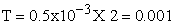The frequency is calculated as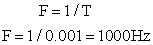The Time/Div was set to 20 µs and the signal span through 0.25 division while the volt/Div was set to 0.5 V.

The period is calculated as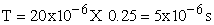The frequency is calculated as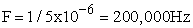The frequency and amplitude of the carrier were held constant while the frequency of the modulating signal was varied. The result obtained is depicted in Table 1 below.

#### Table 1. Varying the modulating signal frequency while carrier frequency remains constant

The frequency and amplitude of the modulating signal were held constant while the carrier frequency was varied. The results obtained were shown in Table 2 below.

#### Table 2. Varying the carrier frequency while the modulating frequency remains constant

The amplitude of both the modulating signal and the carrier signal was varied while the maximum and minimum values of the modulated carrier wave were recorded as in Table 3.

#### Table 3. Maximum and Minimum value of the modulated carrier wave at varied amplitude

Using equation (10), the modulating index at various points was calculated from Table 3 above and different percentages of modulating index were obtained. The various graphs of the experimental results were depicted as follows:

Figure 8. The graph of Output against Mod. signal at constant Carrier signal

### 5. Conclusion

In this paper the performance of modulating scheme has been investigated using square law approach. From the experimental results obtained in the Laboratory, it was observed that the graphs of output against modulating signal and carrier signal keeping one of the parameters constant showed that the output signal at 0.5 ms/div increased as the varying parameter increased and vice-versa.

The experimental results also showed the different modulating index obtained at various values of minimum and maximum values of the carrier signal with the modulating index ranging from 47.4% to 100%. This approach has proved experimentally to be the best way of analysing Amplitude modulating scheme.

### References

  Dmitriev, A.S, Kuzmin, L.V, and Laktushkin A.M, (2009), Amplitude Modulation and Demodulation of Chaotic Signals. Institute of Radio-Engineering and Electronics, Russian Academy of Sciences.In article  I-Hui, H, and Kourosh, S, (2010), Detection of Sinusoidal Amplitude in Logarithmic Frequency sweeps across wide regions of the spectrum. Hearing Reserch Journals, Vol. 262, no. 1-2, pp. 9-18.In article CrossRef  Bowdler, D, (2008), Amplitude Modulation of Wind Turbine Noise. Review of the evidence. Industrial Wind Action Group.In article  Newkirk, D, and Karlquist, R, (2004), Mixers, modulators and demodulators. The ARRL Handbook for Radio Communications, Newington: ARRL. ISBN 0-87259-196-4. de: Amplituden modulation (81st ed.), pp. 15.1-15.36.In article  Patrick D, (2002) Telecommunication Circuit Design, Second Edition. John Wiley & Sons, Inc. ISBNs: 0-471-41542-1 (Hardback); 0-471-22153-8 (Electronic). Pp 64-65.In article  www.wikipedia.com.In article  http://www.encyclopediapro.com/mw/Amplitude_Modulation-modulation/modulation-index-depth.php.In article